• import org.opencv.core.Core; import org.opencv.core.Mat; import org.opencv.highgui.HighGui; import org.opencv.imgcodecs.Imgcodecs... * 高亮图片处理 * */ public class Hightlight_remove { static voi...

import org.opencv.core.Core;
import org.opencv.core.Mat;
import org.opencv.highgui.HighGui;
import org.opencv.imgcodecs.Imgcodecs;

/**
* 高亮图片处理
*
*/
public class Hightlight_remove {

static void highlightRemove(Mat src, Mat dst) {

for (int i = 0; i < src.rows(); i++) {

for (int j = 0; j < src.cols(); j++) {
double B = src.get(i, j);
double G = src.get(i, j);
double R = src.get(i, j);

double alpha_r = R / (R + G + B);
double alpha_g = G / (R + G + B);
double alpha_b = B / (R + G + B);

double alpha = Math.max(Math.max(alpha_r, alpha_g), alpha_b);
double MaxC = Math.max(Math.max(R, G), B);
double minalpha = Math.min(Math.min(alpha_r, alpha_g), alpha_b);
double beta_r = 1 - (alpha - alpha_r) / (3 * alpha - 1);
double beta_g = 1 - (alpha - alpha_g) / (3 * alpha - 1);
double beta_b = 1 - (alpha - alpha_b) / (3 * alpha - 1);
double beta = Math.max(Math.max(beta_r, beta_g), beta_b);
double gama_r = (alpha_r - minalpha) / (1 - 3 * minalpha);
double gama_g = (alpha_g - minalpha) / (1 - 3 * minalpha);
double gama_b = (alpha_b - minalpha) / (1 - 3 * minalpha);
double gama = Math.max(Math.max(gama_r, gama_g), gama_b);

double temp = (gama * (R + G + B) - MaxC) / (3 * gama - 1);

double[] data = new double;
data = B - (temp + 0.5);
data = G - (temp + 0.5);
data = R - (temp + 0.5);
dst.put(i, j, data);
}
}
}

public static void main(String[] args) {
Mat dst = new Mat(src.size(), src.type());
highlightRemove(src, dst);
Imgcodecs.imwrite("D:\\c\\snooker\\chuli.png", dst);
HighGui.imshow("", dst);
HighGui.waitKey();
HighGui.destroyAllWindows();
System.exit(0);
}

}

效果图：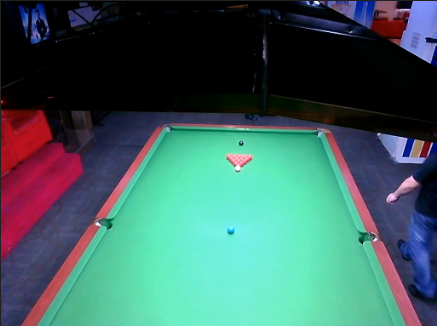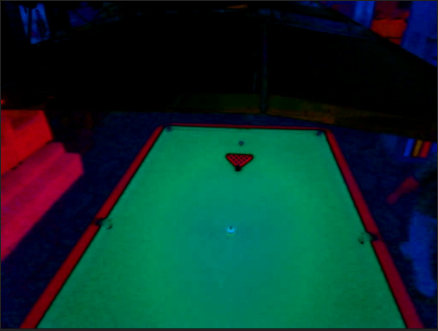参考：http://blog.sina.com.cn/s/blog_6388b0f8010137hy.html
展开全文java
• OpenCV图像操作OpenCVMat数据类型Mat基础操作图像遍历基本遍历指针遍历forEach访问图像像素点像素范围处理空图像创建图像缩放寻找图像像素最大值与最小值计算图像的均值和标准方差convertTo函数cvtColor()函数窗口...


OpenCV图像操作
OpenCVMat数据类型Mat基础操作图像遍历基本遍历指针遍历forEach
访问图像像素点像素范围处理空图像创建图像缩放寻找图像像素最大值与最小值计算图像的均值和标准方差convertTo函数cvtColor()函数
窗口相关利用鼠标点击显示像素值或者三维坐标
图像与绘制轮廓近似取多边形边缘点近似曲线模拟
重要数学操作掩膜操作膨胀、腐蚀、开操作、闭操作------形态学操作腐蚀膨胀开运算闭运算黑帽顶帽梯度操作
滤波边缘提取

OpenCV
OpenCV官方网址. 非常推荐查看官方文档
Mat数据类型
参考网址. cv::Mat 类的对象有一个成员函数 type() 用来返回矩阵元素的数据类型，返回值是 int 类型，不同的返回值代表不同的类型。 ![在这里插入图片描述](https://img-blog.csdnimg.cn/20210119202705346.png?x-oss-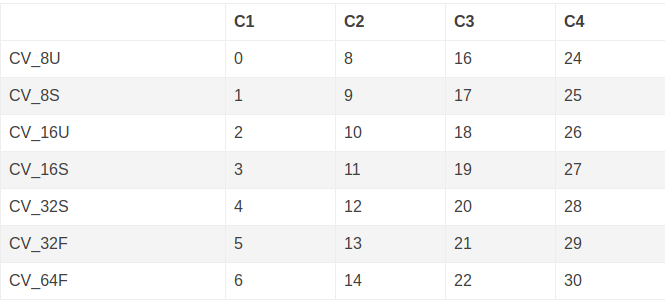表头的 C1, C2, C3, C4 指的是通道(Channel)数。灰度图像只有 1 个通道，是 C1；JPEG格式 的 RGB 彩色图像就是 3 个通道，是 C3；PNG 格式的彩色图像除了 RGB 3个通道外，还有一个透明度通道，所以是 C4。
at 方法接受的是 uchar 这样的数据类型，而非 CV_8U。在已知通道数和每个通道数据类型的情况下，指定给 at 方法的数据类型如下表所示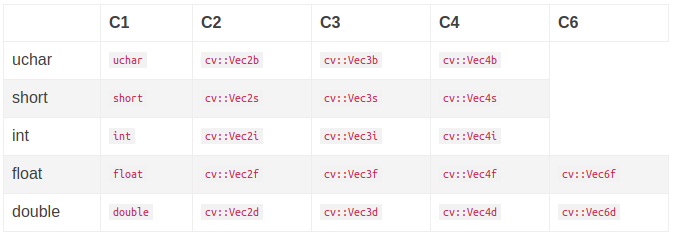Mat基础操作
图像遍历
基本遍历
for (int m = 0; m < pointMat.cols; m++) { // rows为竖向，cols为横向
for (int n = 0; n < pointMat.rows; n++) {
cv::Vec3f point = pointMat.at<cv::Vec3f>(n, m);
}
}

指针遍历


forEach
Mat image = Mat::zeros(3, 3, CV_8UC1);
image.forEach<uchar>([&](uchar& pixel, const int position[]) -> void {
// position 在单通道情况下，是一个包含两个元素的数组，即x, y;
pixel = position + position;
});
cv::Mat A = image; for(int i=0;i<A.rows;i++) { // 下面都是打印用的
for(int j=0;j<A.cols;j++)
cout<<(int)A.at<uchar>(i,j)<<' ';
cout<<endl;
}

访问图像像素点
# 使用 at 方法访问数据元素的时候要指明数据类型
pointMat.at<cv::Vec3f>(n, m)   // 是先横向后竖向
# 获取图像像素指针
Mat.ptr<uchar>(int i=0) /*获取像素矩阵的指针，索引i表示第几行，从0开始计行数。*/
const uchar*  current = myImage.ptr<uchar>(row );/*获得当前行指针*/
p(row, col) = current[col] /*获取当前像素点P(row, col)的像素值*/

像素范围处理
// saturate_cast<uchar> // 防止溢出
saturate_cast<uchar>((g_nContrastValue*0.01)*(g_srcImage.at<Vec3b>(y, x)[c]) + g_nBrightValue)

空图像创建
cv::Mat dispImg(30 + size*h, 100 + size*w, CV_8UC3, Scalar(100, 100, 100));   //需要注意长和宽的区别

图像缩放
renerence–python
cv2.resize(src, dsize[, dst[, fx[, fy[, interpolation]]]])
插值方式有：
cv.INTER_NEAREST	最近邻插值
cv.INTER_LINEAR	双线性插值
cv.INTER_CUBIC	双线性插值
cv.INTER_AREA	使用像素区域关系重新采样。它可能是图像抽取的首选方法，因为它可以提供无莫尔条纹的结果。但是当图像被缩放时，它类似于INTER_NEAREST方法。
resize(imagesource, imagedist, cv::Size(rows, cols));//多用双线性差值实现缩放

resized = cv2.resize(img, None, fx=0.6, fy=0.6, interpolation=cv2.INTER_AREA)
可用于保留宽高比，fx,fy为放大或缩小的系数

寻找图像像素最大值与最小值
参考
void cv::minMaxLoc(InputArray  src, double *   minVal,  double *   maxVal = 0, Point *       minLoc = 0,  Point *       maxLoc = 0, InputArray   mask = noArray()   )
• src：需要寻找最大值和最小值的图像或者矩阵，要求必须是单通道矩阵
• minVal：图像或者矩阵中的最小值。
• maxVal：图像或者矩阵中的最大值。
• minLoc：图像或者矩阵中的最小值在矩阵中的坐标。
• maxLoc：图像或者矩阵中的最大值在矩阵中的坐标。

计算图像的均值和标准方差
cv::Scalar cv::mean(InputArray src,  InputArray mask = noArray() )
src：待求平均值的图像矩阵。
求取的是图像矩阵的每个通道的平均值

同时求取图像每个通道的平均值和标准方差
void cv::meanStdDev(InputArray src,   OutputArray mean,   OutputArray stddev,  InputArray mask = noArray()   )
src：待求平均值的图像矩阵。
• mean：图像每个通道的平均值，参数为Mat类型变量。
• stddev：图像每个通道的标准方差，参数为Mat类型变量。

convertTo函数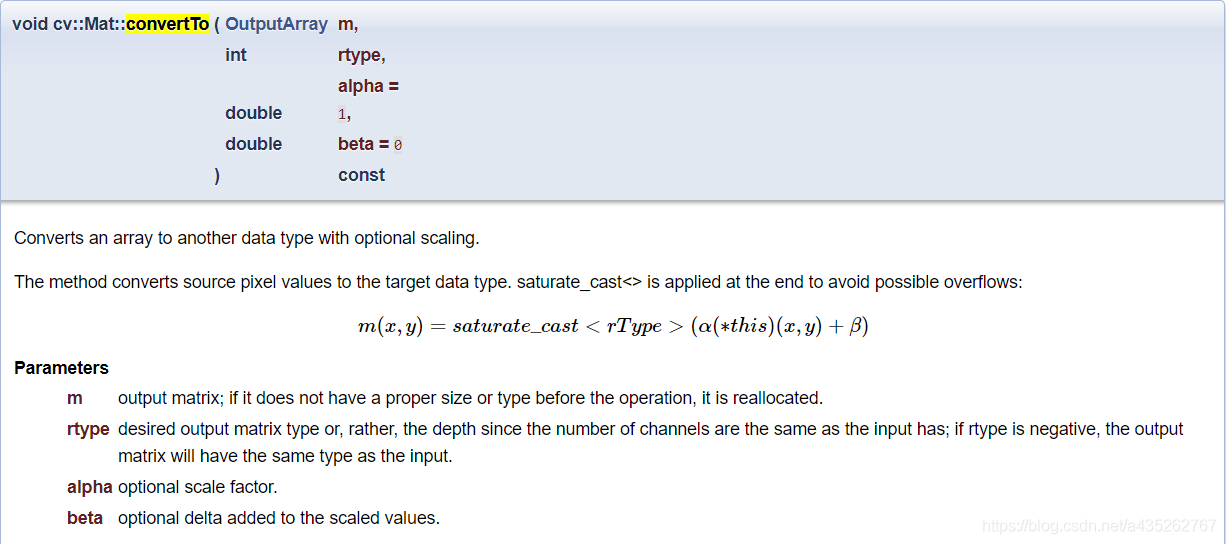• convertTo可以改变Mat的深度，例如可以把Mat的type从CV_8UC3改为CV_16SC3 • convertTo不可以改变Mat的通道数，例如不可以把Mat的type从CV_8UC3改为CV_8UC1，即使你填入的转换类型通道数不同，输出的通道数还是与输入的通道数相同 • convertTo支持就地(in-place)操作
cvtColor()函数
负责转换不同通道的Mat，因为该函数的第4个参数就可以设置目的Mat数据的通道数，一般情况下这个函数是用来进行色彩空间转换的
cvtColor( image, gray_image, COLOR_BGR2GRAY );

窗口相关
getWindowImageRect获取窗口大小

利用鼠标点击显示像素值或者三维坐标
void onmouse(int event, int x, int y, int flags, void *param) {
if (event == cv::EVENT_LBUTTONDOWN) {
cv::Mat pointMat = *((cv::Mat *)param);
std::cout << pointMat.at<cv::Vec3f>(y, x) << std::endl;
// circle(img,Point(x,y),50,Scalar(0,255,0),1);
}
}

cv::namedWindow("image");
setMouseCallback("image", onmouse, &pointMat);
while (true) {
cv::imshow("image", image);
int key = cv::waitKey(20);
if (key == 27) {
break;
}
}

图像与绘制
轮廓近似
approx = cv2.approxPolyDP(contour,epsilon,True)
第一个参数是轮廓的点集。
第二个参数epsilon的含义如下所述，滤掉的线段集离新产生的线段集的距离为d，若d小于epsilon，则滤掉，否则保留。
第三个参数指示新产生的轮廓是否闭合。
返回的是一些列点组成的多边形。

取多边形边缘点
参考
# 可用来检测二值图像的边缘轮廓
findContours(image, mode, method[, contours[, hierarchy[, offset]]]) -> contours, hierarchy

近似曲线模拟
reference
approximate_polygon(coords, tolerance)
# coords: 坐标点序列
# tolerance: 容忍值

重要数学操作
掩膜操作
reference
Mat kernel=(Mat_<char>(3,3)<< 0, -1, 0, -1, 5, -1 , 0, -1, 0);
filter2D( src, dst, src.depth(), kernel );
//输入，输出图像，目标图像深度（如果没写或为-1，将生成与原图像深度相同的图像），卷积核

①提取感兴趣区,用预先制作的感兴趣区掩模与待处理图像相乘,得到感兴趣区图像,感兴趣区内图像值保持不变,而区外图像值都为0。
②屏蔽作用,用掩模对图像上某些区域作屏蔽,使其不参加处理或不参加处理参数的计算,或仅对屏蔽区作处理或统计。
③结构特征提取,用相似性变量或图像匹配方法检测和提取图像中与掩模相似的结构特征。
④特殊形状图像的制作。用选定的图像、图形或物体,对待处理的图像(全部或局部)进行遮挡,来控制图像处理的区域或处理过程。用于覆盖的特定图像或物体称为掩模或模板。
膨胀、腐蚀、开操作、闭操作------形态学操作
reference
void morphologyEx( InputArray src, OutputArray dst,
int op, InputArray kernel,
Point anchor = Point(-1,-1), int iterations = 1,
int borderType = BORDER_CONSTANT,
const Scalar& borderValue = morphologyDefaultBorderValue() )

//获取自定义核 第一个参数MORPH_RECT表示矩形的卷积核，当然还可以选择椭圆形的、交叉型的
Mat kernel = getStructuringElement(MORPH_RECT, Size(18, 18));
morphologyEx(img, out, MORPH_OPEN, kernel);   //输入，输出图像，运算种类，核
这个函数也可实现腐蚀和膨胀，修改第三个参数即可
enum MorphTypes{
MORPH_ERODE    = 0, //腐蚀
MORPH_DILATE   = 1, //膨胀
MORPH_OPEN     = 2, //开操作
MORPH_CLOSE    = 3, //闭操作
MORPH_TOPHAT   = 5, //顶帽操作
MORPH_BLACKHAT = 6, //黑帽操作
MORPH_HITMISS  = 7
};

腐蚀
卷积核沿着图像滑动，如果与卷积核对应的原图像的所有像素值都是1，那么中心元素就保持原来的像素值，否则就变为零。分割(isolate)独立的图像元素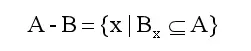图像A用卷积模板B来进行腐蚀处理，通过模板B与图像A进行卷积计算，得出B覆盖区域的像素点最小值，并用这个最小值来替代参考点的像素值。如图所示，将左边的原始图像A腐蚀处理为右边的效果图A-B。
kernel = np.ones((3,3), np.uint8)
detect_res = cv2.erode(detect_res, kernel, iterations = 3)

膨胀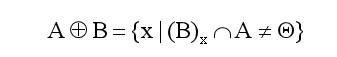图像膨胀是腐蚀操作的逆操作，类似于“领域扩张”，将图像中的高亮区域或白色部分进行扩张，其运行结果图比原图的高亮区域更大，线条变粗了，主要用于去噪。连接(join)相邻的元素
(1) 图像被腐蚀后，去除了噪声，但是会压缩图像。
(2) 对腐蚀过的图像，进行膨胀处理，可以去除噪声，并且保持原有形状。
kernel = np.ones((3,3), np.uint8)
im = cv2.dilate(im, kernel, iterations = 1)

腐蚀、膨胀可用于去噪（低尺寸结构元素的腐蚀操作很容易去掉分散的椒盐噪声点），图像轮廓提取、图像分割、寻找图像中的明显的极大值区域或极小值区域等。
开运算
先腐蚀，后膨胀，将两块连系不紧密的图像区域分开 （１）开运算能够除去孤立的小点，毛刺和小桥，而总的位置和形状不便，可清除一些小东西(亮的)，放大局部低亮度的区域。 （２）开运算是一个基于几何运算的滤波器。 （３）结构元素大小的不同将导致滤波效果的不同。 （４）不同的结构元素的选择导致了不同的分割，即提取出不同的特征。
morphologyEx(img, out, MORPH_OPEN, kernel);//输入，输出图像，运算种类，核

reference
闭运算
闭运算 = 先膨胀运算，再腐蚀运算（看上去将两个细微连接的图块封闭在一起） （1）闭运算能够填平小湖（即小孔），弥合小裂缝，而总的位置和形状不变，可清除小黑点。 （2）闭运算是通过填充图像的凹角来滤波图像的。 （3）结构元素大小的不同将导致滤波效果的不同。 （4）不同结构元素的选择导致了不同的分割。
morphologyEx(img, out, MORPH_CLOSE, kernel);//输入，输出图像，运算种类，核

黑帽
黑帽是闭运算与原图的差值图像，可得到图像内部的小孔，或者前景色中的小黑点，可突出原图像中比周围暗的区域。 黑帽运算(img) = 闭运算图像(img) - 原始图像(img)
dst = cv2.morphologyEx(src, cv2.MORPH_BLACKHAT, kernel)

想获取更多的细节，可以将卷积设置为10*10
顶帽
顶帽是原图像与开运算的差值图像，可突出原图像中比周围亮的区域 顶帽运算(img) = 原始图像(img) - 开运算(img)
kernel = np.ones((10,10), np.uint8)
result = cv2.morphologyEx(src, cv2.MORPH_TOPHAT, kernel)

可以将外部噪声被提取出来，提高内核矩阵kernel可以提取更多元素
梯度操作
膨胀图与腐蚀图之差，保留物体的边缘轮廓
滤波
边缘提取
展开全文c++
• OpenCV图像处理几何变换1 图像缩放2 图像平移3 图像旋转4 仿射变换5 透射变换形态学操作1 连通性2.形态学操作2.1 腐蚀和膨胀2.2 开闭运算2.3 礼帽和黑帽总结 几何变换 1 图像缩放 缩放是对图像的大小进行调整，即使...


OpenCV图像处理
几何变换1 图像缩放2 图像平移3 图像旋转4 仿射变换5 透射变换
形态学操作1 连通性2.形态学操作2.1 腐蚀和膨胀2.2 开闭运算2.3 礼帽和黑帽
总结

几何变换
1 图像缩放
缩放是对图像的大小进行调整，即使图像放大或缩小。 1.API
cv2.resize(src,dsize,fx=0,fy=0,interpolation=cv2.INTER_LINEAR)


参数：
src : 输入图像
dsize: 绝对尺寸，直接指定调整后图像的大小

interpolation：插值方法，

2.示例
import cv2 as cv
# 1. 读取图片
# 2.图像缩放
# 2.1 绝对尺寸
rows,cols = img1.shape[:2]
res = cv.resize(img1,(2*cols,2*rows),interpolation=cv.INTER_CUBIC)

# 2.2 相对尺寸
res1 = cv.resize(img1,None,fx=0.5,fy=0.5)

# 3 图像显示
# 3.1 使用opencv显示图像(不推荐)
cv.imshow("orignal",img1)
cv.imshow("enlarge",res)
cv.imshow("shrink）",res1)
cv.waitKey(0)

# 3.2 使用matplotlib显示图像
fig,axes=plt.subplots(nrows=1,ncols=3,figsize=(10,8),dpi=100)
axes.imshow(res[:,:,::-1])
axes.set_title("绝对尺度（放大）")
axes.imshow(img1[:,:,::-1])
axes.set_title("原图")
axes.imshow(res1[:,:,::-1])
axes.set_title("相对尺度（缩小）")
plt.show()2 图像平移
图像平移将图像按照指定方向和距离，移动到相应的位置。 1.API
cv.warpAffine(img,M,dsize)

参数： img: 输入图像 M： 2*∗3移动矩阵 dsize: 输出图像的大小
2.示例
需求是将图像的像素点移动(50,100)的距离：
import numpy as np
import cv2 as cv
import matplotlib.pyplot as plt
# 1. 读取图像

# 2. 图像平移
rows,cols = img1.shape[:2]
M = M = np.float32([[1,0,100],[0,1,50]])# 平移矩阵
dst = cv.warpAffine(img1,M,(cols,rows))

# 3. 图像显示
fig,axes=plt.subplots(nrows=1,ncols=2,figsize=(10,8),dpi=100)
axes.imshow(img1[:,:,::-1])
axes.set_title("原图")
axes.imshow(dst[:,:,::-1])
axes.set_title("平移后结果")
plt.show()3 图像旋转
图像旋转是指图像按照某个位置转动一定角度的过程，旋转中图像仍保持这原始尺寸。图像旋转后图像的水平对称轴、垂直对称轴及中心坐标原点都可能会发生变换，因此需要对图像旋转中的坐标进行相应转换。 在OpenCV中图像旋转首先根据旋转角度和旋转中心获取旋转矩阵，然后根据旋转矩阵进行变换，即可实现任意角度和任意中心的旋转效果。
1.API
cv2.getRotationMatrix2D(center, angle, scale)


参数： center：旋转中心 angle：旋转角度 scale：缩放比例
返回： M：旋转矩阵 调用cv.warpAffine完成图像的旋转
2.示例
import numpy as np
import cv2 as cv
import matplotlib.pyplot as plt
# 1 读取图像

# 2 图像旋转
rows,cols = img.shape[:2]
# 2.1 生成旋转矩阵
M = cv.getRotationMatrix2D((cols/2,rows/2),90,1)
# 2.2 进行旋转变换
dst = cv.warpAffine(img,M,(cols,rows))

# 3 图像展示
fig,axes=plt.subplots(nrows=1,ncols=2,figsize=(10,8),dpi=100)
axes.imshow(img1[:,:,::-1])
axes.set_title("原图")
axes.imshow(dst[:,:,::-1])
axes.set_title("旋转后结果")
plt.show()4 仿射变换
图像的仿射变换涉及到图像的形状位置角度的变化，是深度学习预处理中常到的功能,仿射变换主要是对图像的缩放，旋转，翻转和平移等操作的组合。
那什么是图像的仿射变换，如下图所示，图1中的点1, 2 和 3 与图二中三个点一一映射, 仍然形成三角形, 但形状已经大大改变，通过这样两组三点（感兴趣点）求出仿射变换， 接下来我们就能把仿射变换应用到图像中所有的点中，就完成了图像的仿射变换。需要注意的是，对于图像而言，宽度方向是x，高度方向是y，坐标的顺序和图像像素对应下标一致。所以原点的位置不是左下角而是右上角，y的方向也不是向上，而是向下。
在仿射变换中，原图中所有的平行线在结果图像中同样平行。为了创建这个矩阵我们需要从原图像中找到三个点以及他们在输出图像中的位置。然后cv2.getAﬃneTransform 会创建一个 2x3 的矩阵，最后这个矩阵会被传给函数 cv2.warpAﬃne。
示例
import numpy as np
import cv2 as cv
import matplotlib.pyplot as plt
# 1 图像读取

# 2 仿射变换
rows,cols = img.shape[:2]
# 2.1 创建变换矩阵
pts1 = np.float32([[50,50],[200,50],[50,200]])
pts2 = np.float32([[100,100],[200,50],[100,250]])
M = cv.getAffineTransform(pts1,pts2)
# 2.2 完成仿射变换
dst = cv.warpAffine(img,M,(cols,rows))

# 3 图像显示
fig,axes=plt.subplots(nrows=1,ncols=2,figsize=(10,8),dpi=100)
axes.imshow(img[:,:,::-1])
axes.set_title("原图")
axes.imshow(dst[:,:,::-1])
axes.set_title("仿射后结果")
plt.show()5 透射变换
透射变换是视角变化的结果，是指利用透视中心、像点、目标点三点共线的条件，按透视旋转定律使承影面（透视面）绕迹线（透视轴）旋转某一角度，破坏原有的投影光线束，仍能保持承影面上投影几何图形不变的变换。
在opencv中，我们要找到四个点，其中任意三个不共线，然后获取变换矩阵T，再进行透射变换。通过函数cv.getPerspectiveTransform找到变换矩阵，将cv.warpPerspective应用于此3x3变换矩阵。
1.示例
import numpy as np
import cv2 as cv
import matplotlib.pyplot as plt
# 1 读取图像
# 2 透射变换
rows,cols = img.shape[:2]
# 2.1 创建变换矩阵
pts1 = np.float32([[56,65],[368,52],[28,387],[389,390]])
pts2 = np.float32([[100,145],[300,100],[80,290],[310,300]])

T = cv.getPerspectiveTransform(pts1,pts2)
# 2.2 进行变换
dst = cv.warpPerspective(img,T,(cols,rows))

# 3 图像显示
fig,axes=plt.subplots(nrows=1,ncols=2,figsize=(10,8),dpi=100)
axes.imshow(img[:,:,::-1])
axes.set_title("原图")
axes.imshow(dst[:,:,::-1])
axes.set_title("透射后结果")
plt.show()形态学操作
1 连通性
在图像中，最小的单位是像素，每个像素周围有8个邻接像素，常见的邻接关系有3种：4邻接、8邻接和D邻接。分别如下图所示：4邻接：像素p(x,y)的4邻域是：(x+1,y)；(x-1,y)；(x,y+1)；(x,y-1)，用N4(p）表示像素p的4邻接
D邻接：像素p(x,y)的D邻域是：对角上的点 (x+1,y+1)；(x+1,y-1)；(x-1,y+1)；(x-1,y-1)，用ND(p）表示像素p的D邻域
8邻接：像素p(x,y)的8邻域是： 4邻域的点 ＋ D邻域的点，用N8(p）表示像素p的8邻域
连通性是描述区域和边界的重要概念，两个像素连通的两个必要条件是：
1.两个像素的位置是否相邻 2.两个像素的灰度值是否满足特定的相 似性准则（或者是否相等
根据连通性的定义，有4联通、8联通和m联通三种。
4联通：对于具有值VV的像素pp和qq，如果qq在集合N4(p）中，则称这两个像素是4连通。
8联通：对于具有值VV的像素pp和qq，如果qq在集合N8(p）中，则称这两个像素是8连通。对于具有值V的像素p和q，如果:
1.q在集合N4(p）中，或
2.q在集合N4(p）中，并且N4（p）与N4( q）的交集为空（没有值V的像素）
则称这两个像素是m连通的，即4连通和D连通的混合连通。2.形态学操作
形态学转换是基于图像形状的一些简单操作。它通常在二进制图像上执行。腐蚀和膨胀是两个基本的形态学运算符。然后它的变体形式如开运算，闭运算，礼帽黑帽等。
2.1 腐蚀和膨胀
腐蚀和膨胀是最基本的形态学操作，腐蚀和膨胀都是针对白色部分（高亮部分）而言的。
膨胀就是使图像中高亮部分扩张，效果图拥有比原图更大的高亮区域；腐蚀是原图中的高亮区域被蚕食，效果图拥有比原图更小的高亮区域。膨胀是求局部最大值的操作，腐蚀是求局部最小值的操作。
1.腐蚀
具体操作是：用一个结构元素扫描图像中的每一个像素，用结构元素中的每一个像素与其覆盖的像素做“与”操作，如果都为1，则该像素为1，否则为0。如下图所示，结构A被结构B腐蚀后：腐蚀的作用是消除物体边界点，使目标缩小，可以消除小于结构元素的噪声点。 API：
   cv.erode(img,kernel,iterations)

参数：
img: 要处理的图像 kernel: 核结构 iterations: 腐蚀的次数，默认是1
2.膨胀
具体操作是：用一个结构元素扫描图像中的每一个像素，用结构元素中的每一个像素与其覆盖的像素做“与”操作，如果都为0，则该像素为0，否则为1。如下图所示，结构A被结构B腐蚀后：作用是将与物体接触的所有背景点合并到物体中，使目标增大，可添补目标中的孔洞。
API：
   cv.dilate(img,kernel,iterations)

参数：
img: 要处理的图像
kernel: 核结构
iterations: 腐蚀的次数，默认是1
示例 我们使用一个5*5的卷积核实现腐蚀和膨胀的运算：
import numpy as np
import cv2 as cv
import matplotlib.pyplot as plt
# 1 读取图像
# 2 创建核结构
kernel = np.ones((5, 5), np.uint8)

# 3 图像腐蚀和膨胀
erosion = cv.erode(img, kernel) # 腐蚀
dilate = cv.dilate(img,kernel) # 膨胀

# 4 图像展示
fig,axes=plt.subplots(nrows=1,ncols=3,figsize=(10,8),dpi=100)
axes.imshow(img)
axes.set_title("原图")
axes.imshow(erosion)
axes.set_title("腐蚀后结果")
axes.imshow(dilate)
axes.set_title("膨胀后结果")
plt.show()2.2 开闭运算
开运算和闭运算是将腐蚀和膨胀按照一定的次序进行处理。 但这两者并不是可逆的，即先开后闭并不能得到原来的图像。
1.开运算 开运算是先腐蚀后膨胀，其作用是：分离物体，消除小区域。特点：消除噪点，去除小的干扰块，而不影响原来的图像。2.闭运算 闭运算与开运算相反，是先膨胀后腐蚀，作用是消除/“闭合”物体里面的孔洞，特点：可以填充闭合区域。3.API
cv.morphologyEx(img, op, kernel)

参数：
img: 要处理的图像 op: 处理方式：若进行开运算，则设为cv.MORPH_OPEN，若进行闭运算，则设为cv.MORPH_CLOSE Kernel： 核结构
4.示例
使用10*10的核结构对卷积进行开闭运算的实现。
import numpy as np
import cv2 as cv
import matplotlib.pyplot as plt
# 1 读取图像
# 2 创建核结构
kernel = np.ones((10, 10), np.uint8)
# 3 图像的开闭运算
cvOpen = cv.morphologyEx(img1,cv.MORPH_OPEN,kernel) # 开运算
cvClose = cv.morphologyEx(img2,cv.MORPH_CLOSE,kernel)# 闭运算
# 4 图像展示
fig,axes=plt.subplots(nrows=2,ncols=2,figsize=(10,8))
axes[0,0].imshow(img1)
axes[0,0].set_title("原图")
axes[0,1].imshow(cvOpen)
axes[0,1].set_title("开运算结果")
axes[1,0].imshow(img2)
axes[1,0].set_title("原图")
axes[1,1].imshow(cvClose)
axes[1,1].set_title("闭运算结果")
plt.show()2.3 礼帽和黑帽
1.礼帽运算
原图像与“开运算“的结果图之差，如下式计算：因为开运算带来的结果是放大了裂缝或者局部低亮度的区域，因此，从原图中减去开运算后的图，得到的效果图突出了比原图轮廓周围的区域更明亮的区域，且这一操作和选择的核的大小相关。
礼帽运算用来分离比邻近点亮一些的斑块。当一幅图像具有大幅的背景的时候，而微小物品比较有规律的情况下，可以使用顶帽运算进行背景提取。
2.黑帽运算
为”闭运算“的结果图与原图像之差。数学表达式为：黑帽运算后的效果图突出了比原图轮廓周围的区域更暗的区域，且这一操作和选择的核的大小相关。
黑帽运算用来分离比邻近点暗一些的斑块。
3.API
cv.morphologyEx(img, op, kernel)

参数：
img: 要处理的图像
op: 处理方式：4.示例
import numpy as np
import cv2 as cv
import matplotlib.pyplot as plt
# 1 读取图像
# 2 创建核结构
kernel = np.ones((10, 10), np.uint8)
# 3 图像的礼帽和黑帽运算
cvOpen = cv.morphologyEx(img1,cv.MORPH_TOPHAT,kernel) # 礼帽运算
cvClose = cv.morphologyEx(img2,cv.MORPH_BLACKHAT,kernel)# 黑帽运算
# 4 图像显示
fig,axes=plt.subplots(nrows=2,ncols=2,figsize=(10,8))
axes[0,0].imshow(img1)
axes[0,0].set_title("原图")
axes[0,1].imshow(cvOpen)
axes[0,1].set_title("礼帽运算结果")
axes[1,0].imshow(img2)
axes[1,0].set_title("原图")
axes[1,1].imshow(cvClose)
axes[1,1].set_title("黑帽运算结果")
plt.show()

总结
图像缩放：对图像进行放大或缩小
cv.resize()
图像平移：
指定平移矩阵后，调用cv.warpAffine()平移图像
图像旋转：
调用cv.getRotationMatrix2D获取旋转矩阵，然后调用cv.warpAffine()进行旋转
仿射变换：
调用cv.getAffineTransform将创建变换矩阵，最后该矩阵将传递给cv.warpAffine()进行变换
透射变换：
通过函数cv.getPerspectiveTransform()找到变换矩阵，将cv.warpPerspective()进行投射变换
金字塔
图像金字塔是图像多尺度表达的一种，使用的API：
cv.pyrUp(): 向上采样
cv.pyrDown(): 向下采样 连通性 邻接关系：4邻接，8邻接和D邻接
连通性：4连通，8连通和m连通
形态学操作
腐蚀和膨胀：
腐蚀：求局部最大值
膨胀：求局部最小值
开闭运算：
开：先腐蚀后膨胀
闭：先膨胀后腐蚀
礼帽和黑帽：
礼帽：原图像与开运算之差
黑帽：闭运算与原图像之差
展开全文计算机视觉 深度学习 tensorflow 神经网络
• ## OpenCV图像直方图

千次阅读 2019-03-28 08:28:53
OpenCV图像直方图 微信公众号：幼儿园的学霸 个人的学习笔记，关于OpenCV,关于机器学习, …。问题或建议，请公众号留言; 灰度直方图(Histogram)是数字图像处理中最简单、最有用的工具之一，它概括了一幅图像的灰度...
OpenCV图像直方图

微信公众号：幼儿园的学霸 个人的学习笔记，关于OpenCV,关于机器学习, …。问题或建议，请公众号留言;

灰度直方图(Histogram)是数字图像处理中最简单、最有用的工具之一，它概括了一幅图像的灰度级内容此处对直方图的数学原理以及OpenCV中的示例进行了展示。
目录

文章目录
OpenCV图像直方图目录定义直方图的绘制直方图的性质直方图的应用OpenCV计算并绘制灰度直方图函数声明代码演示
扩展直方图均衡化直方图规范化

定义
灰度直方图（histogram）是灰度级的函数，描述的是图像中每种灰度级像素的个数，反映图像中每种灰度出现的频率。横坐标是灰度级，纵坐标是灰度级出现的频率（对数字图像来说，意味着该灰度级像素的个数）。 [外链图片转存失败,源站可能有防盗链机制,建议将图片保存下来直接上传(img-aCUnuoVx-1611394568988)(https://s2.ax1x.com/2019/03/05/kjGWm4.png)]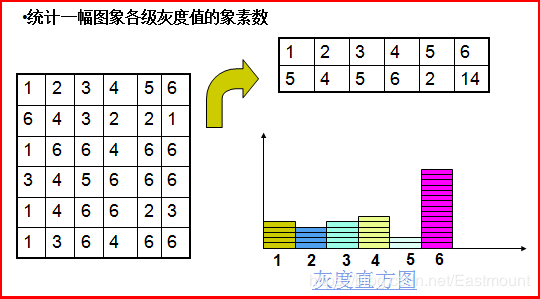过通常会将纵坐标归一化到[0,1]区间内，也就是将灰度级出现的频率（像素个数）除以图像中像素的总数。
对于连续图像，平滑地从中心的高灰度级变化到边缘的低灰度级。直方图定义为：

H

(

D

)

=

lim

⁡

Δ

D

→

0

A

(

D

)

−

A

(

D

+

Δ

D

)

Δ

D

=

−

d

Δ

D

A

(

D

)

−

−

−

−

−

(

1

)

H(D) = \lim_{\Delta D \rightarrow0}\frac{A(D)-A(D+\Delta D)}{\Delta D} = -\frac{d}{\Delta D}A(D)-----(1)

其中

A

(

D

)

A(D)

为阈值面积函数：为一幅连续图像中被具有灰度级D的所有轮廓线所包围的面积。 上式说明，一幅连续图像的直方图是其面积函数的导数的负值。 若将图像看成是二维随机变量，则面积函数相当于其累计分布函数，灰度直方图相当于其概率密度函数。
根据上式介绍的对直方图的定义，对公式进行积分：

∫

D

∞

H

(

D

)

d

D

=

[

−

A

(

D

)

]

D

∞

=

A

(

D

)

\int^\infty_D H(D)dD = [-A(D)]^\infty_D = A(D)

即为图像总面积。 对于离散图像：

∑

0

255

=

N

L

∗

N

S

=

t

h

e

.

t

o

t

a

l

.

p

i

x

e

l

′

s

.

o

f

.

i

m

a

g

e

\sum^{255}_0 = NL * NS = the.total.pixel's.of.image

即为图像总的像素数。
回到式(1),对于离散函数，固定

Δ

D

\Delta D

为1，则：

H

(

D

)

=

A

(

D

)

−

A

(

D

+

1

)

H(D)=A(D)-A(D+1)

显然：对数字图像，任一灰度级D的面积函数就是大于或等于灰度级D的像素的个数。灰度直方图的计算公式如下：

p

(

r

k

)

=

n

k

M

∗

N

p(r_k) = \frac{n_k}{M*N}

其中，

r

k

r_k

是像素的灰度级，

n

k

n_k

是具有灰度级

r

k

r_k

的像素的个数，

M

,

N

M,N

是图像中总的像素个数。
直方图的绘制
1) 将图像的灰度归一化 若图像的灰度级为0,1,……,L-1,令

r

k

=

k

L

−

1

,

k

=

0

,

1

,

⋯

,

L

−

1

r_k = \frac{k}{L-1},k=0,1,\cdots,L-1

则

0

≤

r

k

≤

1

0≤r_k≤1

,L为灰度级的层数，

Δ

L

k

=

r

k

+

1

−

r

k

\Delta L_k = r_{k+1}-r_k

为灰度间隔。 2)计算各灰度级的像素频数（或概率） 设

n

k

n_k

表示灰度级为

r

k

r_k

的像素的个数，

N

N

为总的像素个数，计算像素频数的公式为：

p

r

(

r

k

)

=

n

k

N

p_r(r_k) = \frac{n_k}{N}

3)作图 建立直角坐标系，横轴表示灰度级

r

k

r_k

的取值，纵轴表示灰度级对应的概率

p

r

(

r

k

)

p_r(r_k)

下面为对一幅10X10的图像求其8级灰度直方图的示例 [外链图片转存失败,源站可能有防盗链机制,建议将图片保存下来直接上传(img-rkZgcZhF-1611394568996)(https://s2.ax1x.com/2019/03/06/kvS3VA.png)]
直方图的性质
1）图像被缩减成直方图后，所有的空间信息都丢失了，也就是说，直方图不能提供像素在图像中的位置信息。因此不同的图像可以有相同的直方图。 [外链图片转存失败,源站可能有防盗链机制,建议将图片保存下来直接上传(img-6Q7jMY3i-1611394568997)(https://s2.ax1x.com/2019/03/05/kj1rY6.png)]
2）如果一幅图像包含一个灰度均匀一致的物体，且背景与物体的对比度很强，规定物体的边界时由灰度级$D_1$定义的轮廓线。 [外链图片转存失败,源站可能有防盗链机制,建议将图片保存下来直接上传(img-hbWRkxJH-1611394568999)(https://s2.ax1x.com/2019/03/06/kvSoI1.png)] 3)如果一幅图像由几个连接但不交叠（当然也不能有遗漏）的区域组成，每个区域的直方图已知，则整个图像的直方图是该几个区域的直方图之和。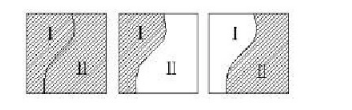直方图的应用
在使用轮廓线确定物体边界时，通过直方图更好的选择边界阈值，进行阈值化处理；对物体与背景有较强对比的景物的分割特别有用；简单物体的面积和综合光密度IOD可以通过图像的直方图求得。 1)数字化参数 可用来判断一幅图像是否合理地利用了全部被允许的灰度级范围。一幅图像应利用几乎全部的灰度级。 [外链图片转存失败,源站可能有防盗链机制,建议将图片保存下来直接上传(img-ESC98TgW-1611394569002)(https://s2.ax1x.com/2019/03/06/kvpAsg.png)] 2)边界阈值选取 1°确立图像中简单物体的边界，用于物体与背景有较强对比时的景物分割 2°阈值化：使用轮廓线作为边界的技术 [外链图片转存失败,源站可能有防盗链机制,建议将图片保存下来直接上传(img-SgFki1Iq-1611394569003)(https://s2.ax1x.com/2019/03/06/kvpJeJ.png)]
OpenCV计算并绘制灰度直方图
函数声明
直方图的计算是很简单的，无非是遍历图像的像素，统计每个灰度级的个数。在OpenCV中封装了直方图的计算函数calcHist，为了更为通用，该函数的参数有些复杂，其声明如下：
calcHist(
const Mat* images,//输入图像的数组，这些图像要有仙童大小、深度（CV_8U,CV_16U,CV_32F）
int images,// 图像数目
const int* channels,// 通道数，要计算的通道数的下标，可以传一个数组 {0, 1} 表示计算第0通道与第1通道的直方图，此数组长度要与histsize ranges 数组长度一致
OutputArray hist,//输出的直方图数据
int dims,// 直方图的维度
const int* histsize,//在每一维上直方图的个数。
//简单把直方图看作一个一个的竖条的话，就是每一维上竖条的个数。
const float* ranges,// 直方图每个维度要统计的灰度级的范围
bool uniform,// true by default 是否归一化
bool accumulate// false by defaut
)


代码演示
下面这段代码展示了利用calcHist函数计算直方图，及动态调整直方图“竖条”的个数的过程。
//====================================================================//
// Created by liheng on 19-3-6.
//Program:图像灰度直方图示例,及动态调整“竖条”数量，观察直方图效果
//Data:2019.3.6
//Author:liheng
//Version:V1.0
//====================================================================//

#include <opencv2/opencv.hpp>

cv::Mat src;//需要计算直方图的灰度图像
cv::Mat histimg;//进行直方图展示的图
cv::MatND hist;//计算得到的直方图结果

int histSize = 50;     // 划分HIST的初始个数，越高越精确

//滚动条函数
void HIST(int t,void*)
{
char string;

if(histSize==0)
{
printf("直方图条数不能为零！\n");
}
else
{
int dims = 1;
float hranges = {0, 255};
const float *ranges = {hranges};   // 这里需要为const类型
int channels = 0;

histimg.create(512,256*4,CV_8UC3);
histimg.setTo(cv::Scalar(0,0,0));

//计算图像的直方图
calcHist(&src, 1, &channels, cv::Mat(), hist, dims, &histSize, ranges);    // cv 中是cvCalcHist
//normalize(hist, hist, 0, histimg.rows*0.5, NORM_MINMAX, -1, Mat());//将直方图归一化，防止某一条过高，显示不全

double maxVal = 0;
cv::Point maxLoc;
cv::minMaxLoc(hist, NULL, &maxVal, NULL, &maxLoc);//寻找最大值及其位置

double bin_w =(double) histimg.cols / histSize;  // histSize: 条的个数，则 bin_w 为条的宽度
double bin_u = (double)histimg.rows/ maxVal;  // maxVal: 最高条的像素个数，则 bin_u 为单个像素的高度

// 画直方图
for(int i=0;i<histSize-1;i++)
{
cv::Point p0=cv::Point(i*bin_w,histimg.rows);

float binValue = hist.at<float>(i);           //   注意hist中是float类型
cv::Point p1=cv::Point((i+1)*bin_w,histimg.rows-binValue*bin_u);

cv::rectangle(histimg,p0,p1,cv::Scalar(0,255,0),2,8,0);
}

//曲线形式的直方图
for (int i = 0; i < histSize; i++)
{
cv::line(histimg,
cv::Point(bin_w*i+bin_w/2, histimg.rows-hist.at<float>(i)*bin_u),
cv::Point(bin_w*(i+1)+bin_w/2, histimg.rows-hist.at<float>(i+1)*bin_u),
cv::Scalar(255, 0, 0), 2, 8, 0);//bin_w/2是为了保证折现位于直方图每条的中间位置
}

//画纵坐标刻度（像素个数）
int kedu=0;
for(int i=1;kedu<maxVal;i++)
{
kedu=i*maxVal/10;
sprintf(string,"%d",kedu);//把一个整数转换为字符串
//在图像中显示文本字符串
cv::putText(histimg, string , cv::Point(0,histimg.rows-kedu*bin_u), cv::FONT_HERSHEY_SIMPLEX,0.5,cv::Scalar(0,255,255),1);

cv::line( histimg,cv::Point(0,histimg.rows-kedu*bin_u),cv::Point(histimg.cols-1,histimg.rows-kedu*bin_u),cv::Scalar(0,0,255));
}
//画横坐标刻度（像素灰度值）
kedu=0;
for(int i=1;kedu<256;i++)
{
kedu=i*20;
sprintf(string,"%d",kedu);//把一个整数转换为字符串
//在图像中显示文本字符串
putText(histimg, string , cv::Point(kedu*(histimg.cols / 256),histimg.rows), cv::FONT_HERSHEY_SIMPLEX,0.5,cv::Scalar(0,255,255),2);
}

cv::imshow( "Histogram", histimg );
}
}

int main( int argc, char** argv )
{

cv::namedWindow( "src", 1);
cv::imshow( "src", src);
cv::namedWindow( "Histogram", 1 );

int maxvalue = 256;
cv::createTrackbar( "histSize", "src", &histSize, maxvalue, HIST );
HIST(0,0);
cv::waitKey(0);

cv::destroyWindow("src");
cv::destroyWindow("Histogram");

return 0;
}

运行效果如下： [外链图片转存失败,源站可能有防盗链机制,建议将图片保存下来直接上传(img-SdkKCJ7o-1611394569004)(https://s2.ax1x.com/2019/03/06/kvujOA.png)]
[外链图片转存失败,源站可能有防盗链机制,建议将图片保存下来直接上传(img-i6GKgPkP-1611394569005)(https://s2.ax1x.com/2019/03/06/kvKB1e.png)]
扩展
利用直方图，还可以对图像进行直方图均衡化和规范化的操作。
直方图均衡化
大多数自然图像，其灰度分布集中在较窄的区间，引起图像细节不够清晰，采用直方图修正后可使图像的灰度间距拉开或使灰度分布均匀，从而增大反差，使图像细节清晰，达到增强的目的。例如一幅过曝光的图片，其灰度级都集中在高亮度范围内，而曝光不足的图片，其灰度集中在低亮度范围内，具有这样直方图的图片其可视效果比较差。 直方图均衡化方法的基本思想是，对在图像中像素个数多的灰度级进行展宽，而对像素个数少的灰度级进行缩减。从而达到清晰图像的目的，增强图像的整体对比度。 其基本处理思路是，把原始图像的灰度直方图从比较集中的某个灰度区间变成在全部灰度范围内的均匀分布。即在每个灰度级上都具有相同像素点数的过程。
直方图规范化
直方图均衡化的优点是能自动增强整个图像的对比度，但它的具体增强效果不易控制，处理的结果总是得到全局的均衡化的直方图。实际工作中，有时需要变换直方图使之成为某个特定的形状，从而有选择地增强某个灰度值范围内的对比度，这时可采用比较灵活的直方图规定化方法。 直方图规范化是指将一幅图像通过灰度转换后，使其具有特定的直方图形式，如使图像与某一标准图像具有相同的直方图，或使图像具有某一特定函数形式的直方图。

下面的是我的公众号二维码图片，欢迎关注。展开全文• 点上方蓝字Python人工智能与深度学习社区获取更多干货在右上方···设为星标★，与你不见不散本文为学习笔记记录本文是《OpenCV实战从入门到精通》系列之第2篇OpenCV | O...计算机视觉 微软 ai 人工智能
• 快速上手OpenCV图像处理图像显示图像腐蚀图像模糊Canny边缘检测 在写作之时，笔者已经接触OpenCV大概三个月了，从刚开始配置环境的“艰辛”，到能用OpenCV完成一些视觉类的小工程，这一路上遇到了不少坑，也是很后悔...计算机视觉 c++
• ## opencv图像处理总结

千次阅读 2016-03-17 14:26:29
opencv图像处理基本操作 1. 矩阵数据类型 通用矩阵数据类型： CV_(S|U|F)C 其中，S表示带符号整数； U表示无符号整数； F表示浮点数； 例如：CV_8UC1　表示8位无符号单通道矩阵； 　CV_32FC2 表示32位...
• OpenCV图像处理 本章的主要内容， 包括如下： 图像的几何变换 图像的形态学转换 图像的平滑方法 直方图的方法 边缘检测的方法 模板匹配和霍夫变换的应用 1 几何变换 学习目标 掌握图像的缩放，平移，旋转等 ...python 人工智能
• 就是原图中高亮部分被腐蚀。 腐蚀的作用 1，边缘检测。 2，噪声滤除。 3，形态骨架提取。 def erode_demo(image): #图像腐蚀 gray = cv.cvtColor(image,cv.COLOR_BGR2GRAY) ret,binary=cv.threshold(gray,0,255,cv...计算机视觉 python 边缘检测
• OpenCV编程实例代码》各章重点知识点简述 第一章 OpenCv环境配置 主要讲解了 OpenCV 的各种开发环境的配置，其中以Sublime 作为主要的配置环境的介绍，这里我们主要使用 VScode 进行开发。 第二章 ...计算机视觉 目标检测 人脸检测识别
• 本专栏主要介绍如果通过OpenCv-Python进行图像处理，通过原理理解OpenCv-Python的函数处理原型，在具体情况中，针对不同的图像进行不同等级的、不同方法的处理，以达到对图像进行去噪、锐化等一系列的操作。...计算机视觉 python
• 图像二值化操作 两种方法，全局固定阈值二值化和局部自适应阈值二值化 全局固定阈值很容易理解，就是对整幅图像都是用一个统一的阈值来进行二值化； 局部自适应阈值则是根据像素的邻域块的像素值分布来确定该像素...
• Qt+opencv图像腐蚀与膨胀概述膨胀腐蚀 概述 形态学操作就是基于形状的一系列图像处理操作。通过将 结构元素 作用于输入图像来产生输出图像。 最基本的形态学操作有二：腐蚀与膨胀(Erosion 与 Dilation)。 腐蚀和膨胀...qt
• 在上一节中，已经完成了OPENCV的配置，在本节接触几个Opencv图像处理相关的程序，看看opencv用简洁的代码能够实现哪些有趣的图像效果。 1、第一个程序：图像显示 #include<opencv2/opencv.hpp> using ...
• 图像膨胀就是图像中的高亮部分进行膨胀，「领域扩张」，效果图拥有比原图更大的高亮区域。 图像腐蚀就是原图中的高亮部分被腐蚀，「领域被蚕食」，效果图拥有比原图更小的高亮区域。 二值化： 函数原型: 参数说明 ...pytorch 人工智能
• 膨胀就是图像中的高亮部分进行膨胀，“领域扩张”，效果图拥有比原图更大的高亮区域。腐蚀就是原图中的高亮部分被腐蚀，“领域被蚕食”，效果图拥有比原图更小的高亮区域。 原理 按数学方面来说，膨胀或者腐蚀操作...python
• 膨胀就是图像中的高亮部分进行膨胀，“领域扩张”，效果图拥有比原图更大的高亮区域。腐蚀就是原图中的高亮部分被腐蚀，“领域被蚕食”，效果图拥有比原图更小的高亮区域。 膨胀与腐蚀能实现多种多样的功能，主要...计算机视觉
•python 计算机视觉 卷积 人工智能
• 1. #include "ReadIDCard.h" //#include "stdafx.h" #include <iostream> #include <stdio.h>...opencv2/opencv.hpp> #include "opencv2/highgui/highgui.hpp" #include "opencv2/imgp...
• 图像中，最小的单位是像素，每个像素周围有8个临界像素，常见的邻接关系有3种：4邻接、8邻接和D邻接。分别如下图所示： 4邻接：像素p（x，y）的4邻域是：（x+1，y）；（x-1，y）；（x，y+1）；（x，y-1），用N4...
• **膨胀腐蚀是基于高亮部分(白色)操作的，膨胀是对高亮部分进行膨胀，类似“”领域扩张，腐蚀是高亮部分被腐蚀，类似领域被蚕食 膨胀腐蚀的应用和 功能： 消除噪声 分割独立元素或连接相邻元素 寻找图像中的...
• // opencv10_dilate.cpp : 此文件包含 "main" 函数。程序执行将在此处开始并结束。 // #include <iostream> #include <opencv2/opencv.hpp> #include <opencv2/imgproc.hpp> using namespace cv...
• 腐蚀 用图像中的暗色部分"腐蚀"掉图像中的高亮部分shell
• 图像腐蚀与图像膨胀 图像的腐蚀（ Erosion ）和膨胀（ Dilation ）是两种基本的形态学运算，主要用来寻找图像中的极大区域和极小区域。 又出来新名词了：形态学。 简单来讲，图像形态学就是改变图像的形状，举例对于...计算机视觉
• 对于一副图像来说，除去高频就是低频了，也就是边缘以内的内容为低频，而边缘内的内容就是图像的大部分信息，即图像的大致概貌和轮廓，是图像的近似信息。 高频 反之，高频即频率变化快，图像什么时候灰度变化快？...高频信息...